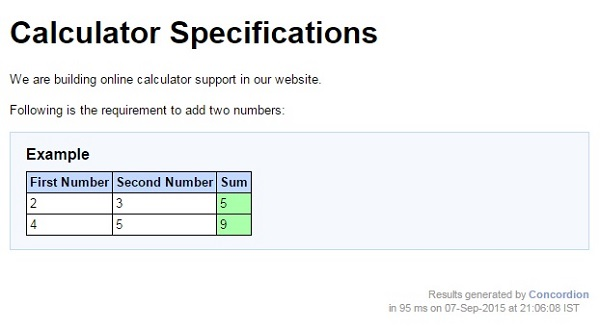# Concordion - Execute on Table

Concordion execute command can be used to run the operation of concordion fixture in a repeating manner. For example, it will be useful if we want to illustrate a requirement with multiple examples in the form of a table.

Consider the following requirement −

```<table>
<tr><th>First Number</th><th>Second Number</th><th>Sum</th></tr>
<tr><td>2</td><td>3</td><td>5</td></tr>
<tr><td>4</td><td>5</td><td>9</td></tr>
</table>
```

If we want to write a specification for a sum function which will accept two numbers and output their sum, then the specification would be as follows −

```<table>
<tr><th>First Number</th><th>Second Number</th><th>Sum</th></tr>
<tr concordion:execute = "#result = sum(#fullName)">
<td concordion:set = "#firstNumber">2</td>
<td concordion:set = "#secondNumber">3</td>
<td concordion:assertEquals = "#result">5</td>
</tr>
<tr concordion:execute = "#result = sum(#fullName)">
<td concordion:set = "#firstNumber">4</td>
<td concordion:set = "#secondNumber">5</td>
<td concordion:assertEquals = "#result">9</td>
</tr>
</table>
```

When Concordion parses the document, it will set a temporary variable #firstNumber to be the value "2" and #secondNumber to be the value "3". Then it will execute the sum() method with parameters as #firstNumber and #secondNumber using execute command and set the result into the #result variable and check that the #result variable is equal to "5". This process is repeated for each table row element.

### Example

Let us have a working Eclipse IDE in place and follow the steps given below to create a Concordion application −

Step Description
1 Create a project with a name concordion and create a package com.tutorialspoint under the src folder in the created project.
2 Add required Concordion libraries using Add External JARs option as explained in the Concordion - First Application chapter.
3 Create Java class System under the com.tutorialspoint package.
4 Create Fixture class SystemFixture under the specs.tutorialspoint package.
5 Create Specification html System.html under the specs.tutorialspoint package.
6 The final step is to create the content of all the Java files and specification file and run the application as explained below.

Here is the content of System.java file −

```package com.tutorialspoint;
public class System {
public int sum(int firstNumber, int secondNumber) {
return firstNumber + secondNumber;
}
}
```

Following is the content of SystemFixture.java file −

```package specs.tutorialspoint;

import org.concordion.integration.junit4.ConcordionRunner;
import org.junit.runner.RunWith;
import com.tutorialspoint.System;

@RunWith(ConcordionRunner.class)

public class SystemFixture {
System system = new System();
public int sum(int firstNumber, int secondNumber) {
return system.sum(firstNumber, secondNumber);
}
}
```

Following is the content of System.html file −

```<html xmlns:concordion = "http://www.concordion.org/2007/concordion">
<link href = "../concordion.css" rel = "stylesheet" type = "text/css" />

<body>
<h1>Calculator Specifications</h1>
<p>We are building online calculator support in our website.</p>
<p>Following is the requirement to add two numbers:</p>

<div class = "example">
<h3>Example</h3>
<table>
<tr>
<th>First Number</th>
<th>Second Number</th>
<th>Sum</th>
</tr>
<tr concordion:execute = "#result = sum(#firstNumber, #secondNumber)">
<td concordion:set = "#firstNumber">2</td>
<td concordion:set = "#secondNumber">3</td>
<td concordion:assertEquals = "#result">5</td>
</tr>
<tr concordion:execute = "#result = sum(#firstNumber, #secondNumber)">
<td concordion:set = "#firstNumber">4</td>
<td concordion:set = "#secondNumber">5</td>
<td concordion:assertEquals = "#result">9</td>
</tr>
</table>
</div>

</body>

</html>
```

Once you are done with creating the source and specification files, let us run the application as JUnit Test. If everything is fine with your application, then it will produce the following result −

```C:\DOCUME~1\ADMINI~1\LOCALS~1\Temp\concordion\specs\tutorialspoint\System.html
Successes: 2, Failures: 0
```

System.html is the output of Concordion test run.